Function Repository Resource:

# HeronFormula

Compute the area of a triangle from the edge lengths

Contributed by: Ed Pegg Jr
 ResourceFunction["HeronFormula"][{a,b,c}] gives the area of the triangle with edge lengths {a,b,c}.

## Details

Heron's formula is also known as Hero's formula.

## Examples

### Basic Examples (2)

A 3-4-5 right triangle has area 6:

 In:=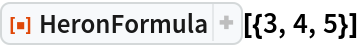Out=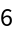A 13-14-15 triangle has area 84:

 In:=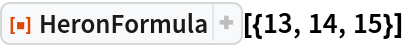Out=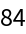### Scope (2)

HeronFormula works for inexact side lengths:

 In:=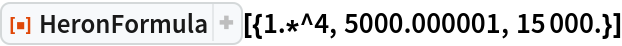Out=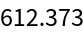HeronFormula works for symbolic arguments:

 In:=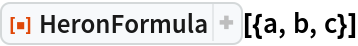Out=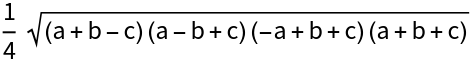### Properties and Relations (2)

Side lengths corresponding to a degenerate triangle will give a zero result:

 In:=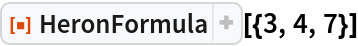Out=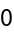If the triangle inequality is not satisfied, HeronFormula remains unevaluated:

 In:=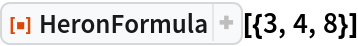Out=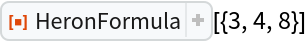In:=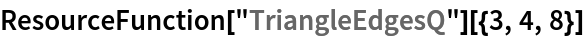Out=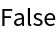## Requirements

Wolfram Language 11.3 (March 2018) or above

## Version History

• 2.0.0 – 31 August 2021
• 1.0.0 – 25 January 2019